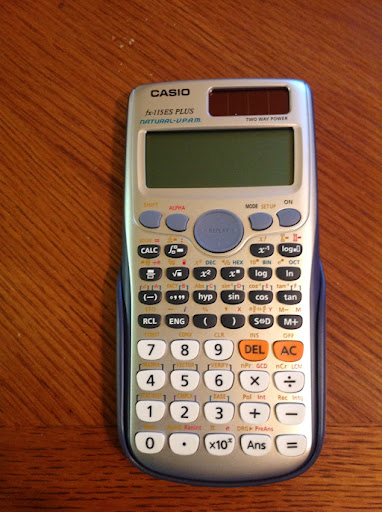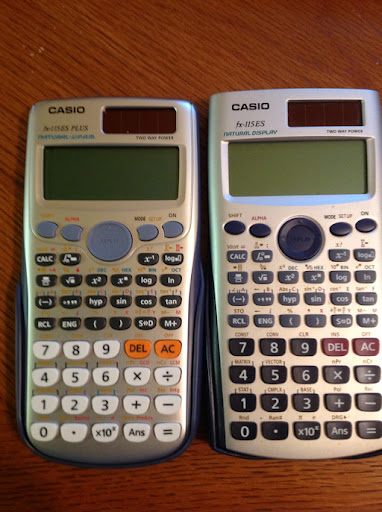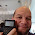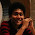## Saturday, April 21, 2012

### Review: Casio fx-115 ES PLUS Review

Welcome to blog entry #73. Just an announcement:

Starting Monday on April 23, 2012, I begin my Calculus Revisited Series, where we revisit some of the major topics of calculus. Each blog entry is going to contain a quick summary of the topic, followed by a few example problems. Hopefully this serves as a review for students in calculus in time for finals or perhaps you want to revisit some of the topics. It will be one blog each day, Monday through Thursday except on Friday, where you get three for the price of one. (don't worry, it's free).

Onto today's topic... the Casio fx-115ES Plus Calculator Review!The fx-115ES PLUS is the successor to the 2006(?) [when was this calculator first released?] fx-115 ES. Outside of the United States, these calculators are called fx-991ES PLUS and fx-991 ES, respectively.

I purchased the fx-115ES PLUS at a WalMart in Glendora, CA. Apparently, this is the only major store at this time that carries the new fx-115ES PLUS outside of ordering online. It cost me \$17.48, around the same price the classic fx-115 ES cost.

The picture below is a comparison between both models. The new fx-115 is on the left.Features

The fx-115ES PLUS retains the Natural-V.P.A.M. (Visually Perfect Algebraic Method), which means that you can enter equations and expressions exactly as written. The MathIO method allows the user to accomplish just that. Fractions, radicals, expressions with π, and calculus function templates are all there. Exact answers can include fractions, square roots, and coefficients of π (MathIO).

For those who want a older style, one-line entry method, the LineIO method has been retained. Personally, I prefer the MathIO method.

All of the other features that were present in the classic fx-115 ES are here to:
* Integrals of f(x)
* Numeric Derivatives: d/dx
* Sums of a function: ∑ f(x)
* Base modes Decimal, Octal, Binary, Hexadecimal
* Numeric solver, of equations and roots of expressions. Yes, the variable to solve for is X. (see below)
* CALC button allows for calculating expressions repeated amount of times.
* Statistics including 1-variable, linear regression (a+bx), quadratic regression (a+bx+cx^2), cubic regression (a+bx+cx^2+dx^3), 2 types of exponential (a + b * e^x and a x^b), power (b a^x), logarithmic (a + b ln x), and inverse (a + b/x).
* Equations - 2x2 and 3x3 simultaneous equations, quadratic, and cubic equation
* Matrices: functions include transpose, inverse, and determinant
* Vectors
* Multi Line Statements with the colon (:)
* Complex Number Mode - unfortunately it's still limited to arithmetic, x^2, 1/x.

Hint: To clear calculator history, while in COMP mode, press the [ ON ] key.

Hint: To force approximate answers, press [SHIFT] [ = ]. This works on both versions of the fx-115 ES.

What's New?
* The number of available memories have increased from 7 to 9. (A, B, C, D, X, Y, M, and now E, F). Previously E and F were available only for the Hexadecimal mode.
* You now have the ability to calculate using repeated numbers. For example, you can type the decimal form of 1/3 using 0.3 with the bar above the three. I believe that this is first line of calculators that has this ability.
* New number functions are: GCD, LCM, Integer Part, Fractional Part, Random Integers, Integer Division (÷R) that gives quotient and remainder, and Prime Factorization (up to three digit factors). To factor a number, enter it, press [ = ], then [SHIFT], [ º ' '' ].
* Products of function f(x): ∏ f(x).
* In Table Mode you can include two functions f(x) and g(x). This is turned on in SET UP.
* The [ALPHA] key acts as both as an variable key and a second shift key.
* The rref and ref functions are added to the Matrix Mode (but not eigenvalues).
* The fx-115ES PLUS has a curve design, and boasts a faster processor.

New Modes
* Inequality Solver of quadratic and cubic equations
* Verification mode, used for compare expressions (i.e. Does π/4 < π/2? Does 1 = 9/9 = e^0?)
* Distribution mode: Normal Distribution (CDF, PDF, and Inverse (Yes!)), Binomial Distribution (CDF, PDF), and Poisson Distribution (CDF, PDF).

Final Thoughts
If the fx-115 ES was solid, then the fx-115ES PLUS is taking a great calculator and making it better. At about \$18, you get a huge bang for the buck.

Rating: ***** (5 out of 5)

------------------------------------------------------------------------
UPDATE-CORRECTION

Many thanks to jcroot for pointing this out to me:

The Solve feature can solve for more than just X. You can specify the variable as so:

equation, variable to solve for

If there is no second argument, then the variable to solve for defaults to X.

Example:
X - 2Y = 1, X solves for X
X - 2Y = 1, Y solves for Y
-------------------------------------------------------------------------

This blog is property of Edward Shore. © 2012

1.Hi there, thank you for this review. I've got a few questions about this one (fx 115 ES plus) and (fx 991 ES plus) as you mentioned they are for different regions only.
But if you look closely, you can see the difference between those two. The biggest problem is that in CASIO website (USA) there isn't any information about fx 115 ES plus, there is only photo and written: "Coming Soon!".
And what concerns me the most is whether fx 115 es plus is better than fx 991es plus? You can check in casio website (or even yours) that you have more functions (in ALPHA mode, written in red color) than fx 991 es plus.
And on the fx 115 es plus photo (in CASIO USA page) you can see inequality on the main screen. Does that mean that fx 115 has inequality solving capability? And in other websites (or even CASIO website) I can't find a proof that fx 991 es plus has inequality solving capability. Thank you in advance.

2.Justin,
Thanks for the questions. :)

Yes, you are 100% correct about the "Coming Soon!". I can't find the manual online either. No idea why WalMart would carry them ahead of the website. (Test run maybe?) I expect the newer fx-115ES PLUS to be in all the major stores and on the Casio website soon.

I checked out the picture of the fx-991ES PLUS again and it seems like the only thing added was the E and F variables. Either this is an old picture or the fx-115ES PLUS truly has more features. I thought the 991 was equivalent to the 115. Hmmm....

Yes, the Inequality Mode is displayed. That mode can solve inequalities involving quadratic or cubic equations. This is new (not on the "classic" fx-115ES).

Eddie

3.That's probably because they know more about calculators produced for European market. Anyways, I'm looking forward to buy the calculator like yours. :)
And they suggested me buying fx-991DE PLUS, not ES, as it has the capability to solve the inequalities. It would be really nice to get one with capability of solving inequalities for my upcoming math exams. By the way, do you know maybe any store that could ship "fx 115ES plus" to Europe? Thanks again, cheers!

1.Justin,

You can check with WalMart to see if they ship items outside of the United States. However, it seems like the fx-991DE PLUS would be the model to buy - it seems to be functionally equivalent to the fx-115ES PLUS in the U.S.

Hope this helps,

Eddie

2.The fx115es plus is the same as the fx-991es. Stop fretting about it and trying to ship the same thing from America for 100% more price. It's only school by the sound of it, in school you barely get past using the + and - signs. I'm doing engineering university and the fx-991es Plus is fine for me, why not for you?

4.Hello Eddie!

I found your blog while looking for a review. I bought this calculator (Casio 115ES Plus) couple of days ago and so far everything works great but I was just wondering if by any chance do you know if this calculator is capable of solving the value of X and Y using the "Solve" button? Or perhaps I am not operating the calculator the right way?

For example, the equation that I am trying to solve is: 4X^2 + 9Y^2 = 144 but I am only getting the value of X (6) But it would be nice to also have the value of Y. I know that this is possible in my TI-36X Pro.

It will be even nicer if we can get a second solution for the X and Y values (in both calculators Casio 115ES Plus and TI-36X Pro).

One last question: Perhaps somebody asked you this before but if you were to decide between this new Casio FX-11ES Plus and TI-36X Pro, which of them would you choose? and in one line... why?

1.Your second question is a tough one. Since I am not a big fan of pressing one key several times to cycle through functions, I will have to go with the fx-115ES.

5.Hi jcroot!

Rechecking the manual, it is possible to solve for variables other than X on the Casio fx-115. Syntax is: equation, variable to solve for.

Example: 4X^2 + 9Y^2 = 144, Y (to solve for Y) The calculator then prompts for a value for X.

Thank you for pointing this out to me - I will edit this review.

6.Helo Eddie!

I also want to thank you for showing us how to solve for more than one variable other than X, I believe this is a really nice feature of this calculator although I have to admit that the syntax in the TI-36X Pro is perhaps a little more user-friendly.

Anyway, do you know if it possible to get a second solution for the equation using {Solve}? Also, do you believe that this fx-115ES is a more powerful machine then the TI-36X Pro?

Sorry for too many questions hehe.

7.I agree that the 36X Pro is nicer in this regard (solver screen). I also like that you do not have to change to specific modes to use matrices, vectors, and complex numbers.

The SOLVE feature only works for one variable for the fx-115 (and for the 36X Pro). Calculus functions are not allowed in the equation.

Eddie

8.Eddie:

I am back to your blog merely because I have a question regarding a particular problem with this calculator (and others calculators as well).

Hopefully you can help me clear my doubts:

Problem: a^2-5a-6 when a = -2

I stored the value of A (which is -2) into this calculator by using the "STO" button. Then I enter the problem into the calculator as it appears in my text book: a^2-5a-6 and then hit the "equal button" and the result is 8.

Now... the problem occurs when entering the problem with the variable already replaced into the calculator: -2^2-5a-6 the result is: 0

Further explanation:

For some reason the calculator assumes there is a parenthesis sign between the variable "a" when replacing the value of variable A.

In other words, when storing the value of the variable into the calculator and then entering the problem as it appears in the text book: a^2-5a-6 the calculator treats this problem as:
(-2)^2-5a-6 and that is why the result is 8.

1.jcroot, what you started is correct.

The reason why the calculator returns 0 when you type -2^2-5*-2-6 is that functions that come after the input value (x^2, x^3, x!, x^-1), roots, and powers get higher priority than negation. This order of operations applies to most TI and Casio calculators that have equation input.

Eddie

2.Thank you for your reply Eddie but I still don't understand. Let me explain myself.

When storing the value of the variable A (which is -2) into the Calculator (TI and Casio) and then entering the problem as it appears in my text book:

a^2-5a-6

NOT

(a)^2-5a-6

Why the calculator assumes there is a parenthesis between the variable a^2 => (a)^2 ? The parenthesis is what makes the result different.

In other words, if I were to solve the problem: a^2-5a-6 without a calculator I believe the answer should be 0 and not 8, is this correct?

3.jcroot,

I honestly have no idea why parenthesis are assumed in variable use; why the programmers made it so a^2 is not the same as -2^2 (when a = -2).

If you are trying to solve a^2-5a-6 (I am assuming this: a^2-5a-6=0), then a^2-5a-6 = (a - 6)(a + 1) implies that a = 6 or a = -1.

Eddie

9.Hello Eddie!

I am back again to ask you another question regarding this calculator. Hopefully you don't mind!

I know that this Casio has the ability to solve inequalities but i am only able to solve inequalities in the form of ax^2+bx+c and I was just wondering how will I be able to solve: -((3)/(2))x≤6 (negative 3 over 2 times x is less then or equal to 6) Do you have any idea?

1.Since the calculator will not allow a=0 in the INEQ mode, we would have to tackle this problem manually.

So: -3/2 x ≤ 6 implies that x ≥ 6 * -2/3 or x ≥ -4

Eddie

10.eddie how many features this casio 115es plus have

11.Amer, I don't have a specific number. My educated guess is above 300.

12.Hi.
I have both fx-115es+ and fx-991es+.
FX-115ES+ have more functions than fx-991es+
Yhis is fx-991es+ http://www.casio.ru/education/images/nau/fx991esplus_big.jpg
and this is fx-115es+ http://www.schoolmart.com/images/products/detail/fx115es_plus_xlarge.jpg
Fx-115es+ is similar to fx-991de+ http://ecx.images-amazon.com/images/I/71yJsIHzNhL._AA1275_.jpg
Compare this pictures and you'll find difference.

13.Hi eddie can you please tell me how to use derivative and integration function on fx 991es plus + which types of question it can solve

14.Omer,

Thanks for writing.

Derivative - the expression must contain the variable X
Keystrokes:
[SHIFT], [ ∫ ] (d/dx)
Syntax:
Math Input: d/dx ( f(X) ) | x = value
Linear Input: d/dx ( f(x), value )

Example (Math Input):
d/dx (X^2-5X+1) | X=2 returns -1

Integral - the expression must contain the variable X
Keystrokes:
[ ∫ ]
Syntax:
Math Input:
b
∫ f(X) dx
a
Linear Input:
∫ (f(X), a, b)

Example:
3
∫ 3X^2 - 1 dx = 24
0

Solver. The calculator solves for any one variable, for any equation or expression. If an expression, the Solver solves for the roots (zeroes) of the expression.

Steps:
1. Enter the equation or expression. Type a comma after and the variable to solve for. The latter step can be omitted if you are solving for X.
2. Press [SHIFT] [CALC] (SOLVE)
3. Enter a guess and press the equals key, [ = ].

Example:
Solve e^X - X = 2, use 7 as a guess.

1. Type "e^X - X = 2, X". (you can leave out the ",X" since we are solving for X).
2. Press [SHIFT] [CALC] (SOLVE)
3. Enter 7, [ = ].

Result: X = 1.146193221.

Hope this helps,

Eddie

15.Thanks eddie for your quick responce it helped alot

16.Fx991es plus and fx115es plus aren't the same

Just look at their pictures

There isn't [VERIFY] above number 6

Take a deeper look you'll notice the differences

17.Kuwaity26,

I did not realize that there are different versions of the 991-ES Plus. Apparently, the 991-ES Plus C is the same as the 115-ES, but I have not found a picture of this version.

Eddie

18.How to save an actual 'formula', not the answer, on the calculator? For example I need to remember: 3933(1+.05/4)^4(4). By looking at the set up of the problem I can then figure out the answer, but need the formula. Does Casio fx=115ES Plus have memory for this?

19.S Lee,

I don't think there is a way to store a formula on the fx-115 ES.

Eddie

20.Hi, Eddie.... I was deciding between the TI 36X Pro and the 991ES Plus(as it's called in my country). It's a difficult decision, because the TI gives more mathematical functions and greater ease of use when it comes to handling matrices, however it gives computational/calculation errors when handling Pi, and mixed fractions according to online reviews... So, while I was initially partial to the TI 36X Pro, I'm undecided now. Can you help???

21.Kaustav1993,

Sorry for being late with my response.

I don't think you can go wrong with either one, 991ES or 36X, as both give roughly the same amount of features, though I like the interface of the 36X Pro better.

If you decide to get the TI-36X make sure you test it first, because the bug has been corrected in newer production models. I had to push through plastic to make sure the π bug was gone. For that reason, I would not recommend getting a TI-36X Pro online (yet).

Please let me know how it goes,

Eddie

22.Hi Eddie (or other guys). I'm from South Africa and bought the fx-991ES PLUS and have a huge problem that it cannot factor integers into prime numbers. I see at your model there's a 'FACT' function at the 2nd function keys, but not at mine. Do you know of any workaround this calculator has to find prime numbers please? My students need to calculate their prime numbers easily.

1.the FX-991ES PLUS doesn't have that functionality. But the FX-991ES PLUS C does.

23.Sue Heyman:

You may have to exchange your calculator(s) with Casio or the place you bought them. If the FACT function is not present, I not sure if there is a function that acts a work-around.

Sorry.

Eddie

24.Hi Eddie, I recently bought an fx-991ES PLUS. This is a ridiculously basic point, but I noticed it doesn't seem to give a value for pi as you'd expect. If you enter [e] then [=] it gives the numerical value 2.718281828 as you'd imagine. But if you enter [pi] then [=] it just gives [pi] ie the symbol. Isn't this what could be called a glitch?

1.You can get the exact value of PI on 991ES PLUS
press SHIFT then [=] when the output is in symbol form

Enjoy !!

3.141592654

2.Isn't that neat? :)

Eddie

25.Garrulus,

This is not a glitch. The exact value of π is a feature of the fx-991ES.

Eddie

26.It's very difficult to choose between this calculator and the TI 36X Pro :( I'm very undecided :(

1.I know. The good thing is that the fx-115ES and 36X are not expensive, less than \$20 each, so if you want, you could buy both.

27.This is a very good review. You did your homework! I gave it a Google +1. You might like my blog, peexamstudy.blogspot, if you are looking forward to the PE. Best wishes!

1.Man from Modesto,

Thank you - much appreciated!

Eddie

28.Which is better for a high school/university student.... TI-36X Pro vs Casio FX-991ESPlus

1.Robert,

I think both calculators are suitable. It is a question of what you prefer.

Eddie

2.Which one of the two do you prefer?

3.This is a really good question, Robert. Personally I tend to lean towards the 36X Pro because I don't have to change modes to operate matrices and complex numbers.

Eddie

29.Can the Casio FX 115 ES Plus Matrix function be used with complex numbers. I can only get it to work with real numbers. Any ideas?

Gary

1.Gary,

The matrix mode only works with real numbers. If you want to work with complex numbers, you will need to be in Complex Mode and manually type the calculation that is needed. Complex mode for the fx-115ES handles arithmetic, x^2, and x^3 functions.

Eddie

30.I want to perform some complex calculation and want to store only imaginary part in A,B C etc register.
is it possible in fx-991ES PLUS calculator?
How can I do that?

1.Jul,

Yes - since the fx-991 ES is an international equivalent of the fx-115ES (United States).

Imaginary and complex numbers are stored to variables by pressing [SHIFT] [RCL] (STO) {letter}. Make sure that you are in Complex Mode.

Eddie

31.Nice review. neat and clean. appretiate it

32.Thanks for such a knowledgeable post. All the points are very clearly defined. Whole work is appreciable.
compare currency

33.Thanks for sharing this information for currency Currency calculator UK

1.You're welcome. Eddie

34.Thanks for this great blog, Very well & insightful.Compare bank exchange rates

35.This comment has been removed by the author.

36.I'm not sure if this thread is still active but I need help with my calculator. The fx-115es plus.
The = sign cannot be hit multiple times when I do multiple multipications (for interest problems) like regular calculators. Is there something I'm missing? I tried looking through the manual but to no avail. I need help! Thanks :)

1.Can you detail the steps to where you have troubles the equals key? Thanks, Eddie

2.He means like if you hit 5 * 4 = and then = again and again it won't keep multiplying by 4 like on some calculators. the best solution for this is to use the Ans button after making the calculation. ex: 5 = (yes just hit five then equal so 5 is the answer), then Ans * 4 =, at this point you can keep hitting equal to multiply by 4 over and over. Hope that helps Spirit.

37.Hi Edward,
Thanks a lot for your nice article. I had one question on my mind [may be it's not 100% related to your post, sorry for that] is there any way to check that the calculator is original or fake? how can i be sure for buying this http://www.bdshop.com/casio-scientific-calculator-fx-991es-plus and be sure it is original? Thanks for your kind help.

38.Hello Eddie! This is me, JCROOT again!

How are you? I am big fan of your blog and I love calculators.

I don't know if you already know this but there is a new version of the Casio FX-115ES Plus, it is called: Casio Classwiz FX-991EX

I bought this new calculator from Casio but I am kind of disappointed since I believe it is missing some previous features from the FX-115ES Plus such as:

1: The repeating decimal button, the LCM and GCD buttons and the Products of function f(x): ∏ f(x).

It is worth the upgrade? Or those features were removed because they were not so useful?

39.This calculator solves systems of equations 4 variables?

40.41.I am Dr Purva Pius, a private money lender. I give out loans with an interest rate of 3% per annual and within the amount of \$1000.00 to \$500,000,000.00 as the loan offer. 100% Project Funding with secured and unsecured loans are available. We are guaranteed in giving out financial services to our numerous clients all over the world. With our flexible lending packages, loans can be processed and funds transferred to the borrower within the shortest time possible. We operate under clear and understandable terms and we offer loans of all kinds to interested clients, firms, companies, and all kinds of business organizations, private individuals and real estate investors. Just complete the form below and get back to us as we expect your swift and immediate response. EMAIL : {urgentloan22@gmail.com}

### TI-84 Plus CE and CE Python 5.7 update

TI-84 Plus CE and CE Python 5.7 update Texas Instruments has released software updates, 5.7, for both the TI-84 Plus CE and TI-84 Plus CE P...